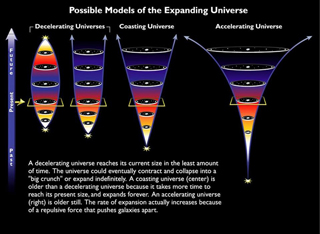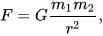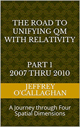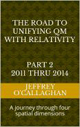# The source of Dark Energy

Dark energy appears to contradict many of our ideas about the way the universe works.  For example, light waves move energy though emptiness of space while Einstein’s equation, E = mc2, tells us that matter and energy are interchangeable, merely different forms of the same thing. However, energy is supposed to have a source either matter or radiation but Dark Energy exists even when space is devoid of all matter and radiation.

For example, Einstein defined the origin mass and its energy of in terms of curvature or displacement in the field properties of space-time not in terms of matter or radiation.   In other words, he defined them as a geometric property of the universe.  Therefore, it is possible that Dark Energy may not be related to matter or radiation but to the geometry of those field properties similar to how he defined matter.Specifically, Einstein defined gravitational energy in terms of positive displacement in the space-time field of our universe which causes mass/energy to become concentrated at the apex of that curvature.  This suggest, since Dark energy is a force that opposes gravity that it could be caused by an opposite directed or negative displacement in geometry of that field.

However, one of the problem integrating Dark Energy into Einstein’s space-time model is the fact that it is causing special expansion of the universe which his space-time model cannot accommodate because it does not include higher spatial dimension for it to expand to.  In other words, because his theories contain only three-spatial dimensions there is no higher spatial one for our universe to expand to.

Granted one can assume that space is expanding relative to itself but that would mean that it must extend to infinity because if it did have a boundary its outer most region would have to be moving with respect to a higher or fourth spatial dimension which would require rewriting his theory to accommodate it.

Additionally, the theoretical model called the Big Bang which most cosmologists accept as the definitive origin for both space and time tell us that it cannot be infinite because it assumes its expansion began not from an infinite volume but form one from within the boundaries of a singularity.

But Einstein provided a solution this problem when defined the magnitude of the gravitational energy in terms of time and the constant velocity of light because that provided a method of converting a unit of time in a space-time environment to unit of space in four *spatial* dimensions.  In other words, because light travel a unique distance for a given time interval it gives us mathematical way to converting a universe composed of four-dimensional space-time to one made up of four *spatial* dimensions.  Additionally, because the velocity of light is constant, he also defined a one to one quantitative and qualitative correspondence between his space-time universe and one made up of four *spatial* dimensions.

The fact that one can use Einstein’s theories to qualitatively and quantitatively derive the *spatial* properties of time in space-time universe in terms of four *spatial* dimensions is one the bases of assuming as was done in the article “Defining energy” Nov 27, 2007 that all forms of energy including gravity and those associated with Dark Energy can be derived in terms of a *spatial* displacement in a "surface" of a three-dimensional space manifold with respect to a fourth *spatial* dimension.

In other words, one can not only use Einstein’s equations to quantitatively and qualitatively derive how both Dark and gravitational energy interacts with time in a space-time dimension but also how it would interact with its *spatial* equivalent in four *spatial* dimensions.

As was mentioned earlier, one problem integrating Dark Energy into Einstein’s space-time model is the fact that it is causing special expansion of three-dimensional space which his model cannot accommodate because it does not include a higher spatial dimension for it to expand towards.  However, redefining his theories in terms its equivalent in four *spatial* dimensions resolves this issue because it defines a higher spatial one in which our three-dimensional space can expand towards.

However, it also provides a logically consistent causality for Dark Energy in terms of his theories since he defined gravity in terms of a depression or positive curvature in a "surface" of a three-dimensional space manifold with respect to fourth *spatial* dimension it would be logical to conclude, due their opposing nature that Dark energy is an elevation or negative curvature in that "surface".

This would also allow one to use Einstein’s theories to understand how Dark Energy is causing the spatial expansion of our universe in terms of either a space-time environment or one made up of fourth *spatial* dimension because as was shown in above these two environments are interchangeable in his theories.

For example, if the walls of an above ground pool filled with water collapse the potential energy associated with the elevated two-dimensional surface of the water will flow or expand and accelerate outward towards the three-dimensional environment sounding it.

However, we know from the study of hydrodynamics that energy "flows" from areas of high density to ones with a low density very similar to how water flows form an elevated or "high density" point to a lower one.

Yet we also know from the observations of the cosmic background radiation that presently our three-dimensional universe has an average energy component equal to about 3.7 degrees Kelvin.

However, if one accepts Einstein’s theories the three-dimensional "surface" of our universe which has an average energy component of 3.7 degree Kelvin one must assume it would be elevated with respect to a fourth *spatial* dimension.

In other words, if the "surface" of a three-dimensional manifold was elevated with respect to a fourth *spatial* dimension by the energy associated with the cosmic background radiation as Einstein tell us must then it would be accelerated outward for the same reason as the water in a pool whose sides had collapsed.

This allows one qualitatively understand the casually of the accelerated expansion of our universe in term of the physical image of water accelerating out of collapsed pool.

The reason why Dark Energy was not the predominant force in the early universe was because its gravitational energy was more concentrated at that time because as the equation definingtells us, its force diminishes with respect to the square of the distance between masses.  Therefore, when the volume of the universe was relatively small its gravitational component determined its expansion rate.  However, as its volume increased Dark Energy took over because the gravitational component of the universe’s mass become less concentrated and therefore weaker with respect it.

Some may feel that this is an over simplification of what appears on the surface to be a rather complex phenomena such as Dark Energy but is no more simplistic than the one used to help us understand how gravity works in a space-time environment.  Granted the math behind this concept may be complex and difficult to understand as it is with the gravitational curvature in space-time.  However, that does not mean that we cannot use it to understand its causality.It should be remembered; Einstein’s genius allows us to choose to define our universe in either a space-time environment or one consisting of four *spatial* dimension when he defined its geometry in terms of the constant velocity of light. This interchangeability broadens the environment encompassed by his theories thereby giving us a new perspective on the how matter energy space and time interact.

Later Jeff

 The Road to Unifying QM with Relativity part 1 2007 thru 2010Ebook \$8.00Paper Back \$15.00 The Road to Unifying QM with Relativity part 2 2011 thru 2014Ebook \$8.00Paper Back \$16.00 The Road to Unifying QM with Relativity part 3 2015 thru 2020Ebook \$8.00Paper Back \$18.00